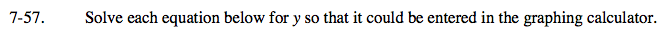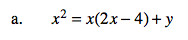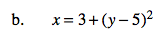### Home > A2C > Chapter 7 > Lesson 7.1.4 > Problem7-57

7-57.First, distribute the x. Then set the equation equal to y and combine like terms.First, subtract 3 from both sides of the equation. Then take the square root of both sides and set the equation equal to y.
Notice there are two results.